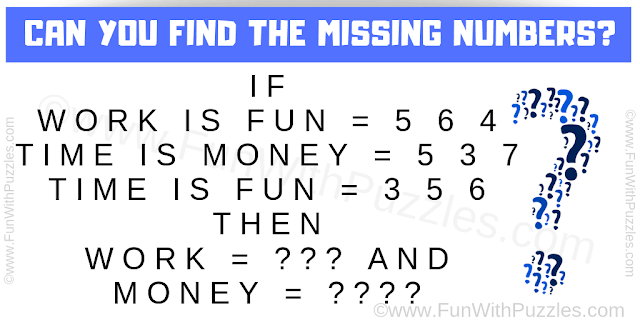This maths logical #reasoning #question is to challenge your brain. In this maths logic puzzle, you are shown some equations. Your challenge is to decipher the logic used in these equations and then find the values of the given variables in these equations. So can you find the value of these missing numbers?Can you solve this Maths Logical Reasoning Question?

Answer of this "Maths Logical Reasoning Question", can be viewed by clicking on the answer button.